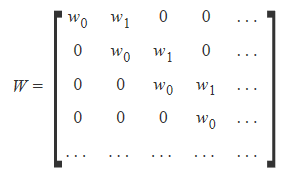# Applying filter to a 3x3 portion of an image

I’m trying to understand how a learned filter is ‘applied’ to a tiny subset of an image. I was referring to one of the readings for this week and specifically looking at the following image:

We start with a 3x3 image on the LHS and ‘apply’ a 3x3 filter on this image. Initially my thought process was that the 3x3 image would be multiplied (matrix multiplication) by the 3x3 filter, resulting in another 3x3 matrix. But looking at this image, we can clearly see that proper matrix multiplication isn’t happening at all. It seems like a piece-wise multiplication where corresponding elements of the 2 matrices are multiplied and then they’re all summed together.

My question is why is this happening? Is there a more intuitive way to understand why this is taking place?

1 Like

Yes that’s exactly what’s happening - it’s a convolution, not a matrix multiplication. You might find this a helpful primer on convolutions: http://colah.github.io/posts/2014-07-Understanding-Convolutions/

1 Like

In the tutorial referenced on convolutions, the following example matrix was used to describe weights of neurons in a layer.I thought the weights of all neurons in a layer were supposed to be the same?

In the tutorial referenced, the analogy was presented of convolutions being like finding total probabilities for an event. However, it became unclear whether the author was trying to relate that concept to why a filter matrix is applied as an element-wise multiplication and then sum, or to the larger picture process of moving a filter across an image (input matrix)?

In a dense layer, the weights between every input and every output is different. In a convolutional layer, the weights for each part of each filter are different, and then they are applied to each part of the image.

I was looking at the same link and using it with top sobel filter. I understand that for every pixel we take all its neighbors (basically a 3 by 3 pixel area around it), multiply it by our filter, sum and the result is the value of the resulting image’s same pixel.

What I have hard time understanding is that if we our top row are positives, middle row are zeros and bottom row is negatives how does adding them result in top edge filter? So basically not getting intuition about how having filter with rows (positives, zeros, negatives) when convoluted on our image makes it a top sobel. Similarly @jeremy mentioned that filter with (negatives, positives, zeros) is top edge filter. I can see the math, verify it and it is doing that but cannot get intuition by which I can look at a filter and be sure that this is right edge filter or something like that

Think about it like this… Under what combinations of pixels will the value of the top sobel filter be at it’s maximum?

+This row should be as high as possible
0 This row is multiplied by 0 so it doesn’t matter

• This row should be as low as possible

If the top and the bottom row are the same they cancel each other out. The further they are apart the higher the output is going to be. Because we’re usually dealing with chars negative numbers will be treated as 0 so it’s a top filter because it has a high value for the top of edges and a low value otherwise.

I understand that for any sobel if we have (+, 0, -) then top row will have maximum value. And when summing top rows effect in the final sum for the pixel will be highest. But doesn’t that hold for each pixel of the image?

Maybe I should check whether my understanding of convolution is correct. Can you check the maths @Even?

Say we have the image of size 3 by 3. We apply zeros padding on sides

0 0 | 0 | 0 0
0 4 | 4 | 4 0
0 1 | 1 | 1 0
0 1 | 1 | 1 0
0 0 | 0 | 0 0

We apply filter
1 | 1 | 1
0 | 0 | 0
-1| -1| -1

Then the image after convolution will be

-2 |-3 |-2
6 | 9 | 6
2 | 3 | 2

If someone looks at this read through the recommended reading at http://colah.github.io/posts/2014-07-Understanding-Convolutions/. Specifically this part helped me greatly. Don’t jump go through the article

We can also detect edges by taking the values −1−1 and 11 on two adjacent pixels, and zero everywhere else. That is, we subtract two adjacent pixels. When side by side pixels are similar, this is gives us approximately zero. On edges, however, adjacent pixels are very different in the direction perpendicular to the edge